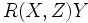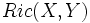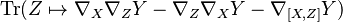# Ricci curvature tensor

Jump to: navigation, search

This article defines a tensor (viz a section on a tensor bundle over the manifold) of type (0,2)

## Description

### Given data

A differential manifold$M$ with a linear connection$\nabla$ on it.

### Definition part

The Ricci curvature tensor is a$(0,2)$-tensor that takes as input two vector fields and outputs a scalar function, as follows.

Let$X$ and$Y$ be two vector fields. Then consider the map that sends a vector field$Z$ to$R(X,Z)Y$ (here$R$ denotes the Riemann curvature tensor).

This is a linear map. The Ricci curvature function$Ric(X,Y)$ is defined as the trace of this map.

Explicitly, it is given by:$\operatorname{Tr}(Z \mapsto \nabla_X\nabla_ZY - \nabla_Z\nabla_XY - \nabla_{[X,Z]}Y)$

The Ricci curvature function is a tensor because the Riemann curvature function is also a tensor.

### For a Riemannian or pseudo-Riemannian manifold

Further information: Ricci curvature tensor of Levi-Civita connection

For a Riemannian manifold or pseudo-Riemannian manifold, we can give the Levi-Civita connection, a natural choice of linear connection. The Ricci curvature tensor of this connection, is termed the Ricci curvature tensor of the Riemannian or pseudo-Riemannian manifold.